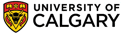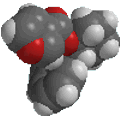Chapter 4: Alcohols and Alkyl Halides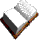What is Hyperconjugation ?

Hyperconjugation is the stabilising interaction that results from the interaction of the electrons in a σ-bond (usually C-H or C-C) with an adjacent empty or partially filled p-orbital or a π-orbital to give an extended molecular orbital that increases the stability of the system.

Based on the valence bond model of bonding, hyperconjugation can be described as "double bond - no bond resonance" but it is not what we would "normally" call resonance, though the similarity is perhaps useful and is shown below.

QUESTION : What is the key difference between hyperconjugation and resonance ? ANSWER

Hyperconjugation is a factor in explaining why increasing the number of alkyl substituents on a carbocation or radical centre leads to an increase in stability.

 Let's consider how a methyl group is involved in hyperconjugation with a carbocation centre.First we need to draw it to show the C-H σ-bonds. Note that the empty p orbital associated with the positive charge at the carbocation centre is in the same plane (i.e. coplanar) with one of the C-H σ-bonds  (shown in blue.)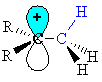This geometry means the electrons in the σ-bond can be stabilised by an interaction with the empty p-orbital of the carbocation centre. (this diagram shows the similarity with resonance and the structure on the right has the "double bond - no bond" character)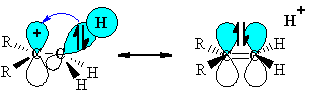• The stabilisation arises because the orbital interaction leads to the electrons being in a lower energy orbital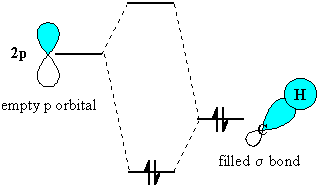• Of course, the C-C σ-bond is free to rotate, and as it does so, each of the C-H σ-bonds in turn undergoes the stabilising interaction.
• The ethyl cation has 3 C-H σ-bonds that can be involved in hyperconjugation.
• The more hyperconjuagtion there is, the greater the stabilisation of the system.
• For example, the t-butyl cation has 9 C-H σ-bonds that can be involved in hyperconjugation.
• Hence (CH3)3C+ is more stable than CH3CH2+
• The effect is not limited to C-H σ-bonds, appropriate C-C σ-bonds can also be involved in hyperconjugation.© Dr. Ian Hunt, Department of Chemistry